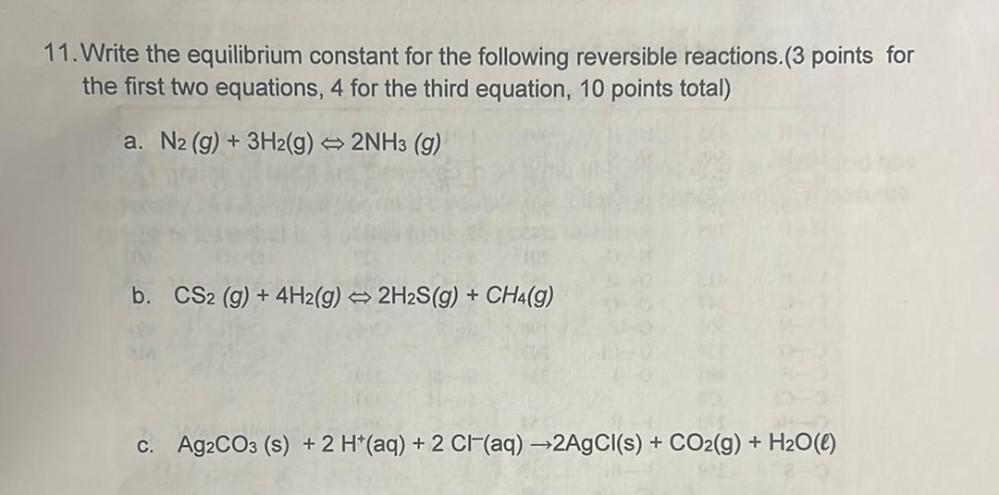Question:

# 11. Write the equilibrium constant for the following reversible reactions.(3 points for the first two equations, 4 for the third11. Write the equilibrium constant for the following reversible reactions.(3 points for the first two equations, 4 for the third equation, 10 points total) a. N2(g) + 3H2(g) 2NH3 (9) b. CS2 (g) + 4H2(g) → 2H2S(9) + CH4(9) + + c. Ag2CO3 (s) + 2 H+(aq) + 2 Cl(aq) +2AgCl(s) + CO2(g) + H2O(C) + 2